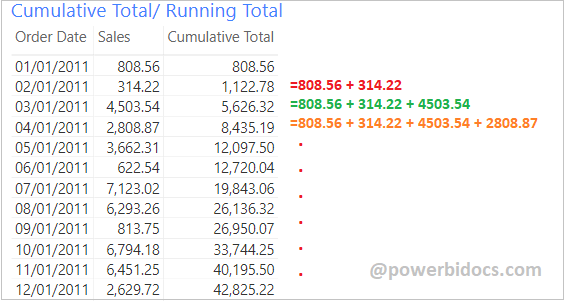Home » DAX » Cumulative Total/ Running Total in Power BI

# Cumulative Total/ Running Total in Power BIThe cumulative or running total is used to watch the summation of numbers that is updated every time when a new number is entered to the sequence.

Refer similar post:

#### Follow these steps in order to create a cumulative total DAX

Step-1: Create a measure with below code

```Cumulative Total =
CALCULATE (
SUM('Global-Superstore'[Sales]),
FILTER (
ALL( 'Global-Superstore'[Order Date] ),
'Global-Superstore'[Order Date] <= MAX ( 'Global-Superstore'[Order Date] )
)
)```

#### Measure Description:

• CALCULATE:  Evaluates an expression in a modified filter context.
• SUM: The SUM function is a aggregation function and it calculates the sum of all numbers in a column.
• FILTER: Returns a table that represents a subset of another table or expression.
• ALL: Returns all the rows in a table, or all the values in a column.
• MAX: Returns the largest value in a column.

Step-2: Now drag the measure into Table visual with some fields and see the output.Cumulative Total in Power BI

#### Another Approach to calculate the cumulative totals:

DATESYTD DAX:

```DATESYTD =

CALCULATE(SUM('Global-Superstore'[Sales]),
DATESYTD('Global-Superstore'[Order Date]))```DATESYTD DAX Running Total

It returns the year wise running total and for every year it will start sales summation from the beginning.

Also you can refer these post in order to calculate cumulative or running total Month, Quarter & Year wise-

Hope you enjoyed the post. Your valuable feedback, question, or comments about this post are always welcome or you can leave us message on our contact form , we will revert to you asap.

## 3 thoughts on “Cumulative Total/ Running Total in Power BI”

1.i believe that there is an error in this example. Since the SUM function needs the [sales] column the ALL function needs to specify the whole table ‘global superstore’ not just the column ‘global superstore'[order date]. e.g.

Cumulative Total =
CALCULATE (
SUM(‘Global-Superstore'[Sales]),
FILTER (
ALL( ‘Global-Superstore’ ),
‘Global-Superstore'[Order Date] <= MAX ( 'Global-Superstore'[Order Date] )
)
)

1.Hi, Filter function needs table name as in first argument. So, we passed ALL with table name and second argument is date column.

2.Hello,

In my sales table I have different products.

How could I do this same query but accumulating product by product?

Thank you